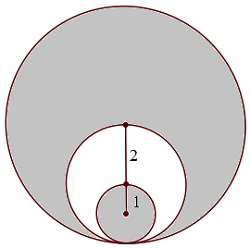# SAT CirclesAs shown in the figure above, the three circles are tangent, the middle circle passes through the center of the big circle, and the small circle passes through the center of the middle circle. What is the area of the shaded region?

(A) $\ \ 6\pi$
(B) $\ \ 11\pi$
(C) $\ \ 13\pi$
(D) $\ \ 19 \pi$
(E) $\ \ 21\pi$

×